# tilenum

Tile location numbers in tiled chart layout

## Syntax

``num = tilenum(t,row,col)``
``num = tilenum(obj)``

## Description

example

````num = tilenum(t,row,col)` returns the tile number for the specified row and column in the tiled chart layout `t`. Specify `row` and `col` scalars or as arrays of row and column numbers.This syntax does not support layouts that use the `"flow"` tile arrangement.```

example

````num = tilenum(obj)` returns the tile numbers for the specified objects in a tiled chart layout. For example, you can use this syntax to get the tile number for a specified axes object.This syntax supports layouts that use any tile arrangement.```

## Examples

collapse all

Create a 2-by-2 tiled chart layout containing four plots.

```t = tiledlayout(2,2); nexttile plot([1 2 3 4]) nexttile scatter(1:7,[10 13 5 33 7 12 9]) nexttile stairs(1:10) nexttile bar(1:5)```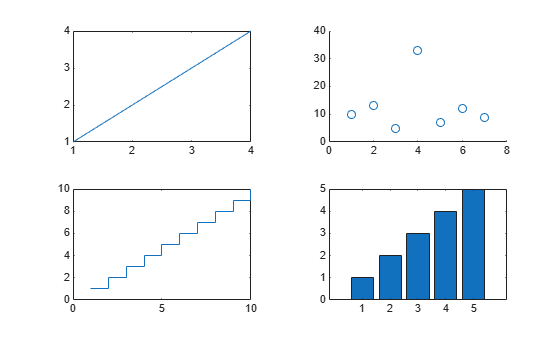Get the number of the tile in the second row and first column.

`num = tilenum(t,2,1)`
```num = 3 ```

Get the tile numbers for all the rows and columns.

`nums = tilenum(t,[1 1 2 2],[1 2 1 2])`
```nums = 1×4 1 2 3 4 ```

Create a 2-by-2 tiled chart layout containing four plots.

```t = tiledlayout(2,2); nexttile plot([1 2 3 4]) nexttile scatter(1:7,[10 13 5 33 7 12 9]) nexttile stairs(1:10) nexttile bar(1:5)```The `Children` property of the `TiledChartLayout` contains all the top-level objects in the layout. In this case, the top-level objects are axes. Note that the objects in the `Children` property are in the opposite order of their creation. To get the tile numbers in the order of creation, call the `flipud` function and pass the reordered array to the `tilenum` function.

`nums = tilenum(flipud(t.Children))`
```nums = 4×1 1 2 3 4 ```

Create a 3-by-3 tiled chart layout containing three plots in the first row. Then create an axes object that spans across the remaining empty tiles (a 2-by-3 region). Create a bar chart in the spanned axes.

```tiledlayout(3,3) nexttile plot([1 2 3 4]) nexttile plot([4 3 2 1]) nexttile stairs(1:10) % Create spanned axes and bar chart spax = nexttile([2 3]); bar(spax,1:10)```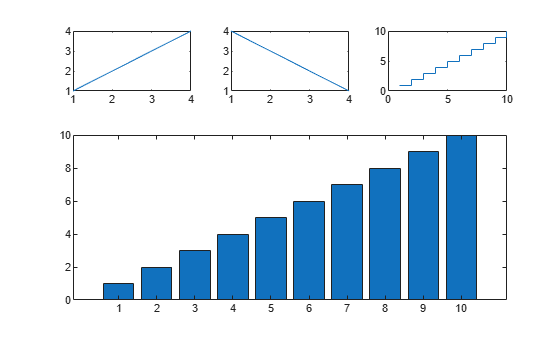Get the tile number for the spanned axes. `tilenum` returns the number of the tile at the upper-left corner of the axes, not the entire set of spanned tiles.

`num = tilenum(spax)`
```num = 4 ```

You can use the `tilenum` function to customize the axes in a particular row or column of the layout's grid. For example, you can locate the axes in the first column or first row, and add labels only to those axes.

Create a grid of 15 plots that show the crop yields of a family farm over five months. Create a 4-by-15 matrix of data. Each column in the matrix contains the data for a particular month.

```scalefactors = (1:15) * 70; data = rand(4,15) .* scalefactors;```

Create string vectors `monthnames` and `crops`, which contain the labels for the left column and top row, respectively. Then create a 5-by-3 tiled chart layout, and plot each column of the data in a separate tile.

```monthnames = ["May" "June" "July" "August" "September"]; crops = ["Asparagus" "Tomatoes" "Lettuce"]; t = tiledlayout(5,3,TileSpacing="compact",Padding="compact"); for i = 1:length(monthnames)*length(crops) nexttile bar(data(:,i)) ylim([0 1000]) end```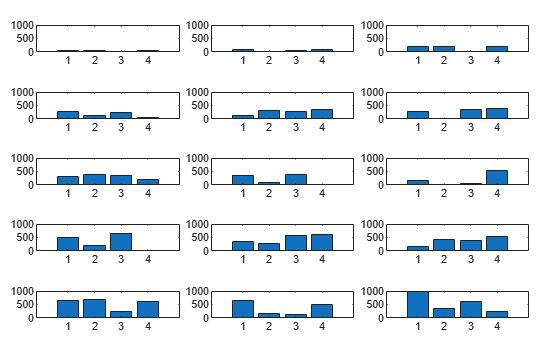Display the month names along the y-axes of the plots in the first column. Use the `Children` property of the `TiledChartLayout` object to get all the axes in the layout but in the opposite order of creation. Call the `flipud` function to reorder the axes.

For each axes you want to add a label to, get the tile number by calling the `tilenum` function. Then index into the `Children` property with the tile number, and pass the resulting axes object to the `ylabel` function along with the month name.

```t.Children = flipud(t.Children); for i = 1:length(monthnames) num = tilenum(t,i,1); ylabel(t.Children(num),monthnames(i)) end```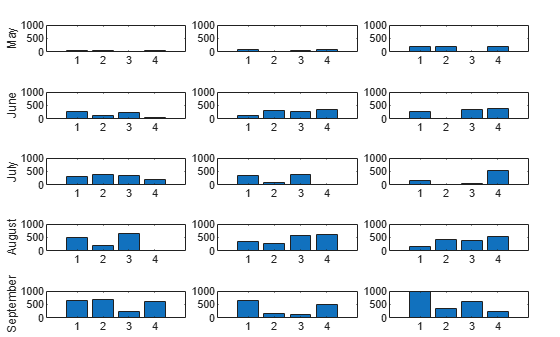Display the crop names as titles of the axes in the first row. Locate and add titles to the appropriate axes by using the `tilenum` function and the `Children` property. Then add an overall title to the layout.

```for i = 1:length(crops) num = tilenum(t,1,i); title(t.Children(num),crops(i)) end title(t,"Johnston Family Farm 2021 Crop Yields")```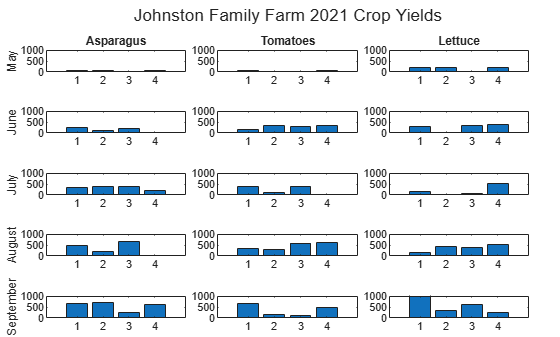## Input Arguments

collapse all

Tiled chart layout containing the tiles you want to locate. Use the `tiledlayout` function to create the `TiledChartLayout` object.

The `TileArrangement` property of the `TiledChartLayout` object must be set to `"fixed"`, which is the default value when you call `tiledlayout(m,n)` to create a fixed number of tiles.

Row numbers, specified as a scalar, vector, or array of positive integer values the same size as `col`. If a row number is greater than the number of rows in the layout, `tilenum` returns `NaN` for that row.

Column numbers, specified as a scalar, vector, or array of positive integer values the same size as `row`. If a column number is greater than the number of columns in the layout, `tilenum` returns `NaN` for that column.

Graphics object or an array of graphics objects that are children of a tiled chart layout. The types of objects you can specify include:

• Axes, such as `Axes`, `PolarAxes`, or `GeographicAxes` objects

• Standalone visualizations, such as `heatmap` charts

• Legends

• Colorbars

• `TiledChartLayout` objects that are nested within the tiles of a tiled chart layout

If a specified object is in one of the `"north"`, `"south"`, `"east"`, or `"west"` tiles, `tilenum` returns `NaN` for that object.

## Output Arguments

collapse all

Tile numbers, returned as a scalar, vector, or array of tile numbers. If you query multiple rows and columns (or multiple objects) by specifying a vector or an array of inputs, `tilenum` returns the output as vectors or arrays of the same size.

`tilenum` returns `NaN` values if you specify a row or column that does not exist, or if `obj` is a graphics object in one of the `"north"`, `"south"`, `"east"`, or `"west"` tiles.

## Version History

Introduced in R2022b